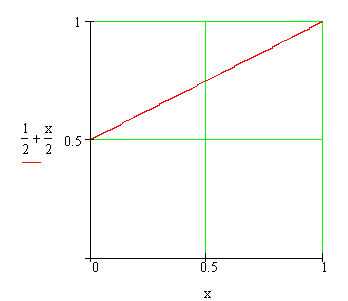Calculus on Demand at Dartmouth College Lecture 24 | Index | Lecture 26 Lecture 25Resources

Math 3 Course Syllabus
Practice Exams

Contents

In this lecture we derive a formula for finding the length of a curve in the plane as an example of modeling using the Method of Accumulations.

Quick Question

What is the length of the slanted line (in red)?Outline

Outline for Arc Length

Arc Length